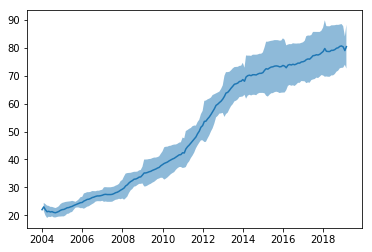{{announcement.body}}
{{announcement.title}}

# Visualizing Trends in a Time Series With Pandas

DZone 's Guide to

# Visualizing Trends in a Time Series With Pandas

### A quick look into how to use the Python language and Pandas library to create data visualizations with data collected from Google Trends.

· Big Data Zone ·
Free Resource

Comment (0)

Save
{{ articles.views | formatCount}} Views

The trend of time series is the general direction in which the values change. In this post, we will focus on how to use rolling windows to isolate it. Let's download the interest in the search term Pancakes from Google Trends and see what we can do with it:

``````import pandas as pd
import matplotlib.pyplot as plt
data = pd.read_csv(url, skiprows=2, parse_dates=['Month'], index_col=['Month'])
plt.plot(data)``````Looking at the data we notice that there's some seasonality (Pancakes Day! Yay!) and an increasing trend. What if we want to visualize just the trend of this curve? We only need to slide a rolling window through the data and compute the average at each step. This can be done in just one line if we use the `rolling` method:

``````y_mean = data.rolling('365D').mean()
plt.plot(y_mean)``````The parameter passed to rolling '365D' means that our rolling window will have a size of 365 days. Check out the documentation of the method to know more.

We can also add highlights to the variation in each year by adding a shade to the chart with the amplitude of the standard deviation:

``````y_std = data.rolling('365D').std()
plt.plot(y_mean)
plt.fill_between(y_mean.index,
(y_mean - y_std).values.T,
(y_mean + y_std).values.T, alpha=.5)``````Warning: the visualization above assumes that the distribution of the data each year follows a normal distribution, which is not entirely true.

Topics:
big data, data visualization python, pandas tutorial, python tutorial

Comment (0)

Save
{{ articles.views | formatCount}} Views

Published at DZone with permission of Giuseppe Vettigli , DZone MVB. See the original article here.

Opinions expressed by DZone contributors are their own.